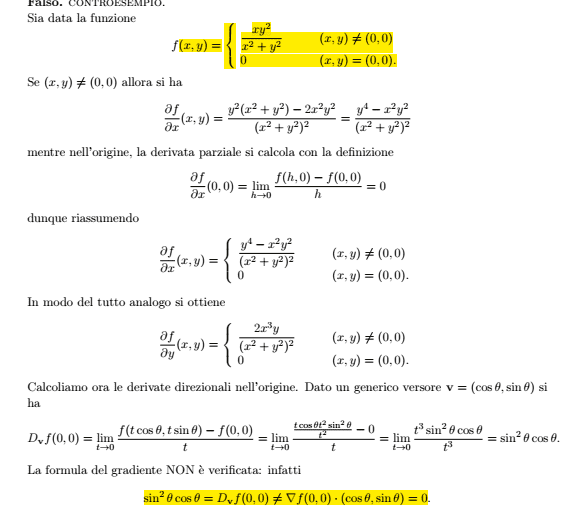# Problem with a directional derivative calculation

Amaelle
Homework Statement:
calculate the directional derivative
Relevant Equations:
directional derivative
Good day

I have a problem regarding the directional derivative (look at the example below)in this example, we try to find the directional derivatives according to the two approaches ( the definition with the limit and the dot product of the vector gradient and the vector direction)
in this example, we got totally different results?

why?

Homework Helper
2022 Award
The partial deivatives are not continuous: for $x= 0$ and $y \neq 0$ you have $\frac{\partial f}{\partial x} = \frac{y^4}{y^4} = 1$ which does not tend to 0 as $y \to 0$.

•PeroK and Amaelle
Amaelle
so If I understand well, the dot product method works only if the function is differentiable? otherwise, we should only use the limit method?

Homework Helper
Gold Member
2022 Award
in this example, we try to find the directional derivatives according to the two approaches ( the definition with the limit and the dot product of the vector gradient and the vector direction)
in this example, we got totally different results?

why?

What your book is doing is calculating the derivative along a parameterised curve through ##(0,0)##:$$g(t) = f(t\cos \theta, t\sin \theta) = t\cos \theta \ \sin^2 \theta$$ Which is well-defined. And, trivially: $$g'(t) = \cos \theta \ \sin^2 \theta$$

Last edited:
•Amaelle
Amaelle
yes thank you, understanding things takes times :) but thank you for easing the pain

•Delta2
Homework Helper
Gold Member
••